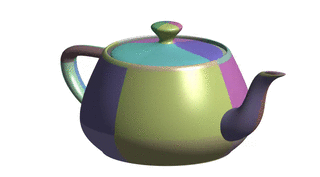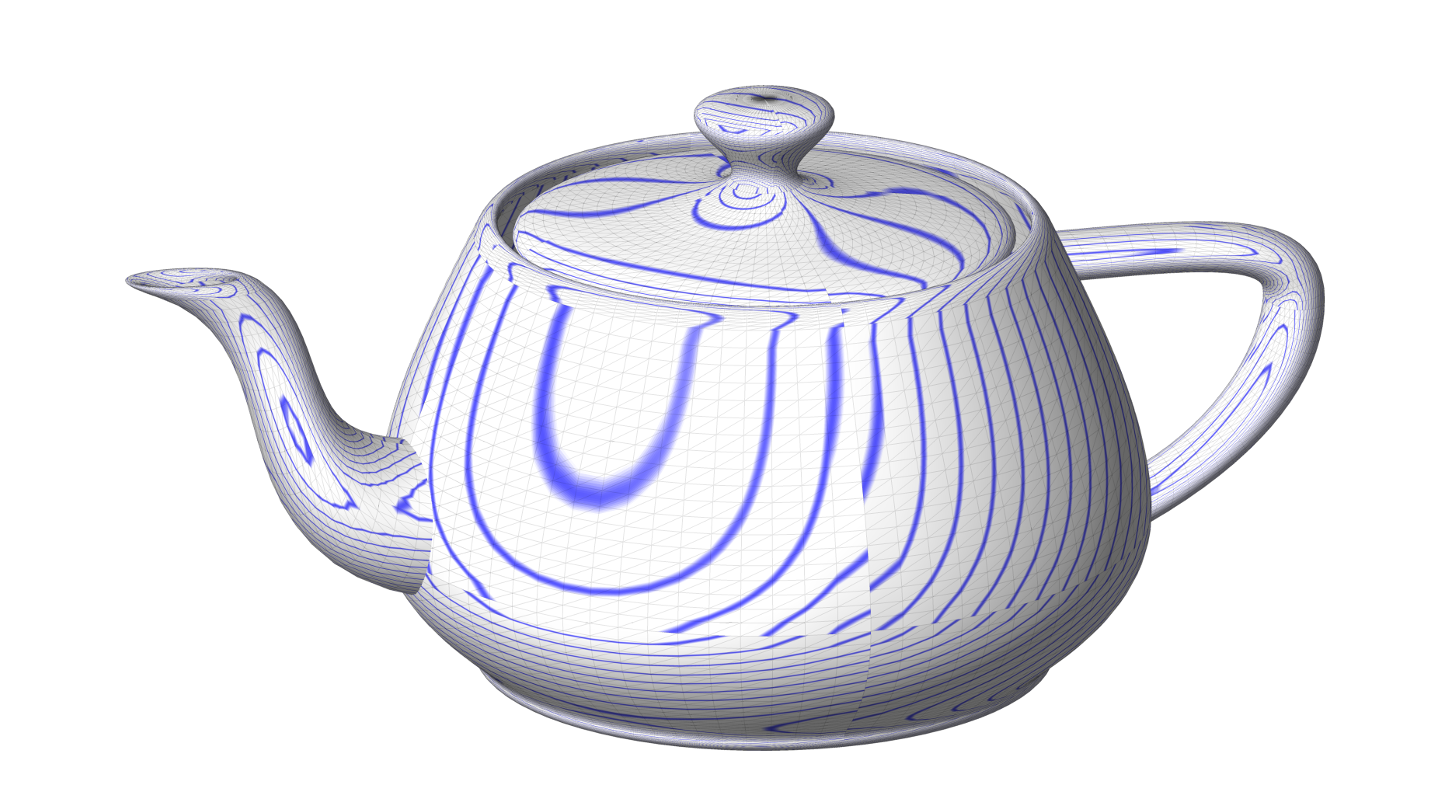# TP6 : Bézier Surfaces

18 March 2016 in

## Code

Do

git pull


or, if you don’t have the local repo,

git clone https://github.com/bbrrck/geo-num-2016.git


As usual, test by

cd TP6/
mkdir build
cd build
cmake ..
make


Today, we start working with surfaces, which means the transition from 2D to 3D. We will use OpenGL for rendering, but don’t worry if you have little or no experience with OpenGL; a wrapper class SimpleViewer is provided. To render a patch, just call

viewer.add_patch(X,Y,Z);


Calling this method repeatedly means adding new patches while keeping the existing ones. Like this, you can render multiple patches at once (i.e. render piecewise Bézier surfaces).

You can also play with settings to set face/edge color:

viewer.set_facecolor( 255, 163,   0 ); // [255,163,  0] = sort of orange
viewer.set_edgecolor(  55,  55,  55 ); // [ 55, 55, 55] = gray


The first build will take a bit longer than usual as there are some new libraries: GLM, GLFW and GLEW; these are all required by the SimpleViewer.

To test the viewer, try running

./geonum_TP6 simple


If everything goes well, you should see a cube. The viewer can be controlled with the mouse: click and drag to rotate, scroll to zoom (also works with pageup/pagedown keys).

Note: If you experience problems compiling the program with all the new libraries, there is an alternative way: use the cmake/CMakeLists2.txt file (copy its contents to ./CMakeLists.txt). In this case, don’t forget to remove/comment #include <SimpleViewer.h>. You can then export your patches via the writePatch(...) function and render them using matplotlib.

## Tensor product Bézier surfaces

Tensor product Bézier surfaces are a direct generalization of Bézier curves. Instead of a control polygon $\mathbf b_i$, we consider a control net $\mathbf b_{ij}$. A Bézier surface is the described as

$S(u,v) = \sum_{i=0}^{m} \sum_{j=0}^{n} \mathbf b_{ij} B_{i}^{m}(u) B_{j}^{n}(v)$

where $m,n$ are degrees in $u,v$, respectively; $B_{l}^{k}$ are Bernstein polynomials.

## Evaluation

To evaluate a point $S(u,v)$ on a surface, let’s fix $j$ and vary $i$.

\begin{equation} \displaystyle B(u,v) = \sum_{j=0}^{n} B_{j}^{n}(v) \underset{= \mathbf b_{j}(u) } { \underbrace{ \left[ \sum_{i=0}^{m} \mathbf b_{ij} B_{i}^{m}(u) \right] } } \end{equation}

The $\mathbf b_{j}(u)$ defines a Bézier curve in $u$ and can be evaluated using the De Casteljau.

\begin{equation} \displaystyle B(u,v) = \sum_{j=0}^{n} \mathbf b_{j}(u)B_{j}^{n}(v) \label{final} \end{equation}

This equation defines a Bézier curve in $v$ with control points $\mathbf b_{j}(u)$ depending on $u$. At the end of the day, we have

• $n+1$ evaluations of the De Casteljau for the degree $m$;
• $1$ evaluation of the De Casteljau for the degree $n$.

Alternatively, we can fix $i$ and vary $j$.Bézier patch evaluation scheme. (image by Pierre-Luc Manteaux)

## Coordinate matrices

In the code, the control points $\mathbf b_{ij}$ are actually stored in three coordinate matrices $\texttt{nX}, \texttt{nY}, \texttt{nZ} \;$ so that

$\mathbf b_{ij}^x = \texttt{nX(i,j)}, \qquad \mathbf b_{ij}^y = \texttt{nY(i,j)}, \qquad \mathbf b_{ij}^z = \texttt{nZ(i,j)}.$

This means the code is more comprehensible as the structure of the matrices directly represents the grid topology of the patch. On the other hand, it also means the computation needs to be done for each coordinate individually.

## Bézier surface vs. piecewise Bézier surface

In practice, a Bézier surface often consists of multiple surface patches, each having its own control polygon. Therefore, it is sometimes called a piecewise Bézier surface.

Some surfaces in the data/ folder consist of more than one patch, ranging from 2 (heart) to 128 (gumbo). They are saved in the BPT format; some datafiles are taken from the website of Ryan Holmes where you’ll also find more details about this format.The discontinuity in isophotes shows the piecewise Bézier Utah teapot is not $\mathcal C^1$.

## ToDo

1. Implement the evaluation of Bézier surfaces for $(u,v) \in [0,1]^2$. For the first tests, use simple and simple2 (as these contain only one patch).
2. When you’re sure the implementation works for the simple cases, test your algorithm on datasets with multiple patches: heart (2), sphere (8), teapot (32) and gumby (128). Don’t set the num_samples parameter too high, always start with smaller values (e.g. 5 or 10).